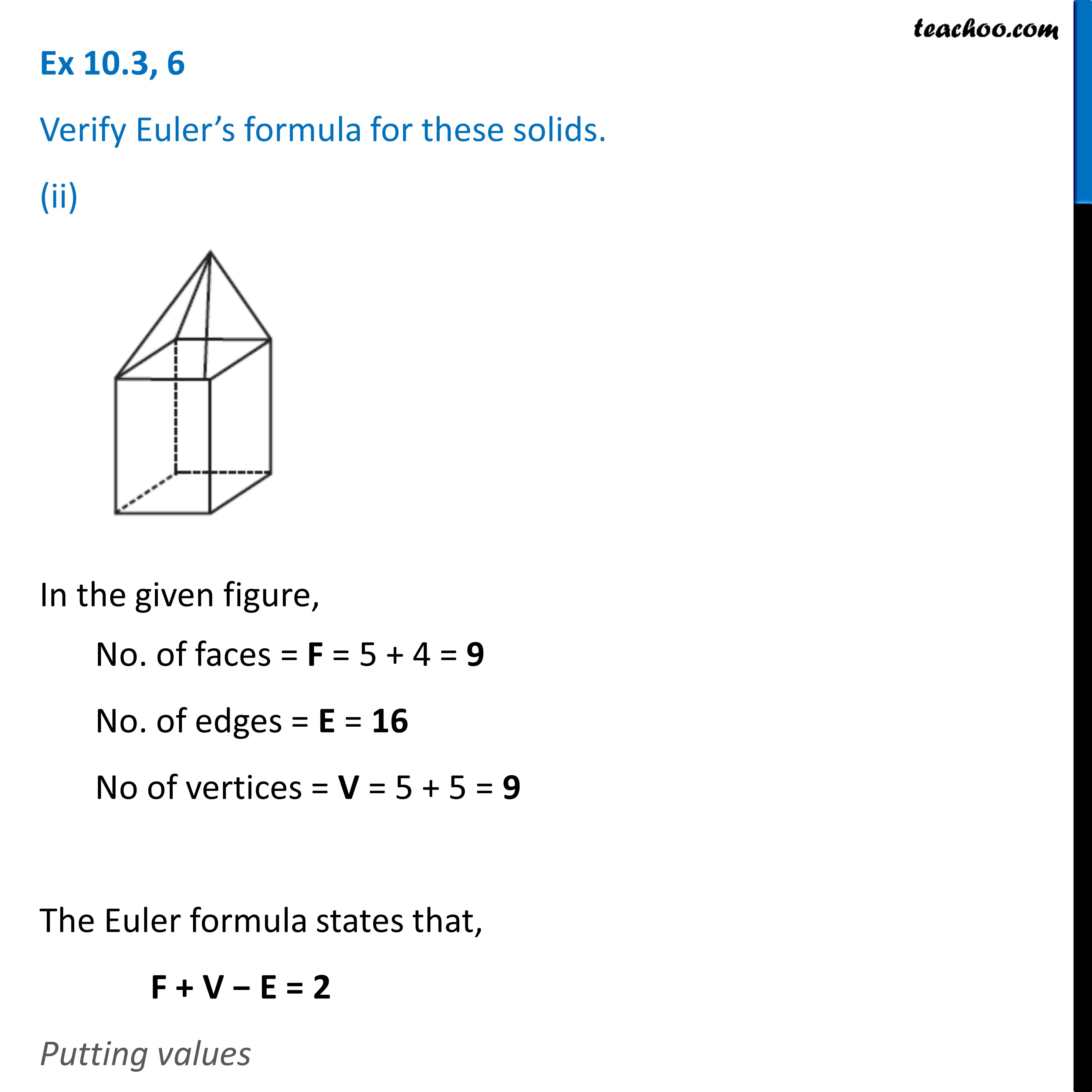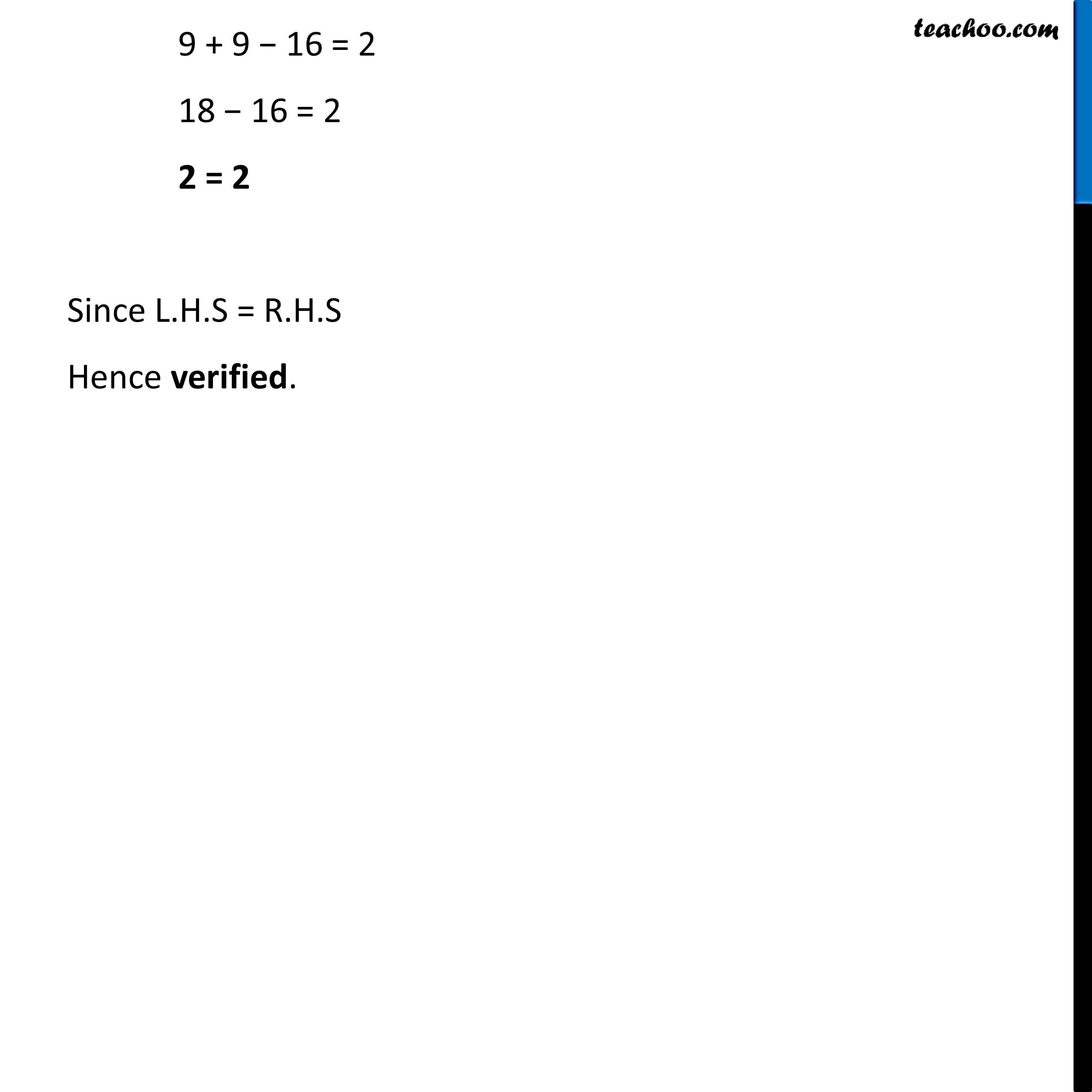Prism and Polyhedrons

Visualising Solid Shapes
Serial order wiseLearn in your speed, with individual attention - Teachoo Maths 1-on-1 Class

### Transcript

Question 6 Verify Euler’s formula for these solids. (ii) In the given figure, No. of faces = F = 5 + 4 = 9 No. of edges = E = 16 No of vertices = V = 5 + 5 = 9 The Euler formula states that, F + V − E = 2 Putting values 9 + 9 − 16 = 2 18 − 16 = 2 2 = 2 Since L.H.S = R.H.S Hence verified.